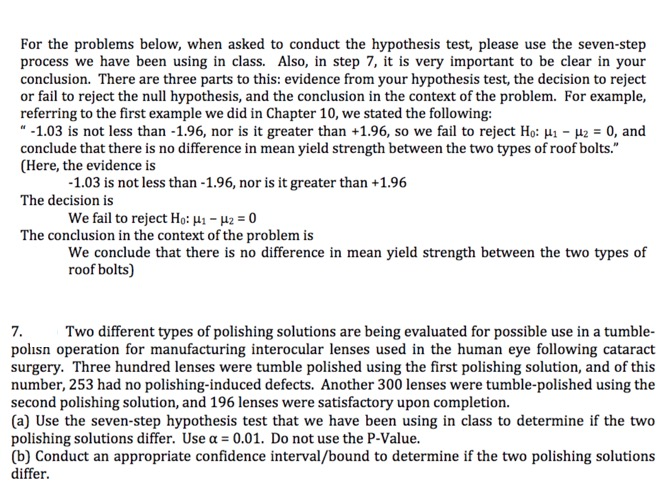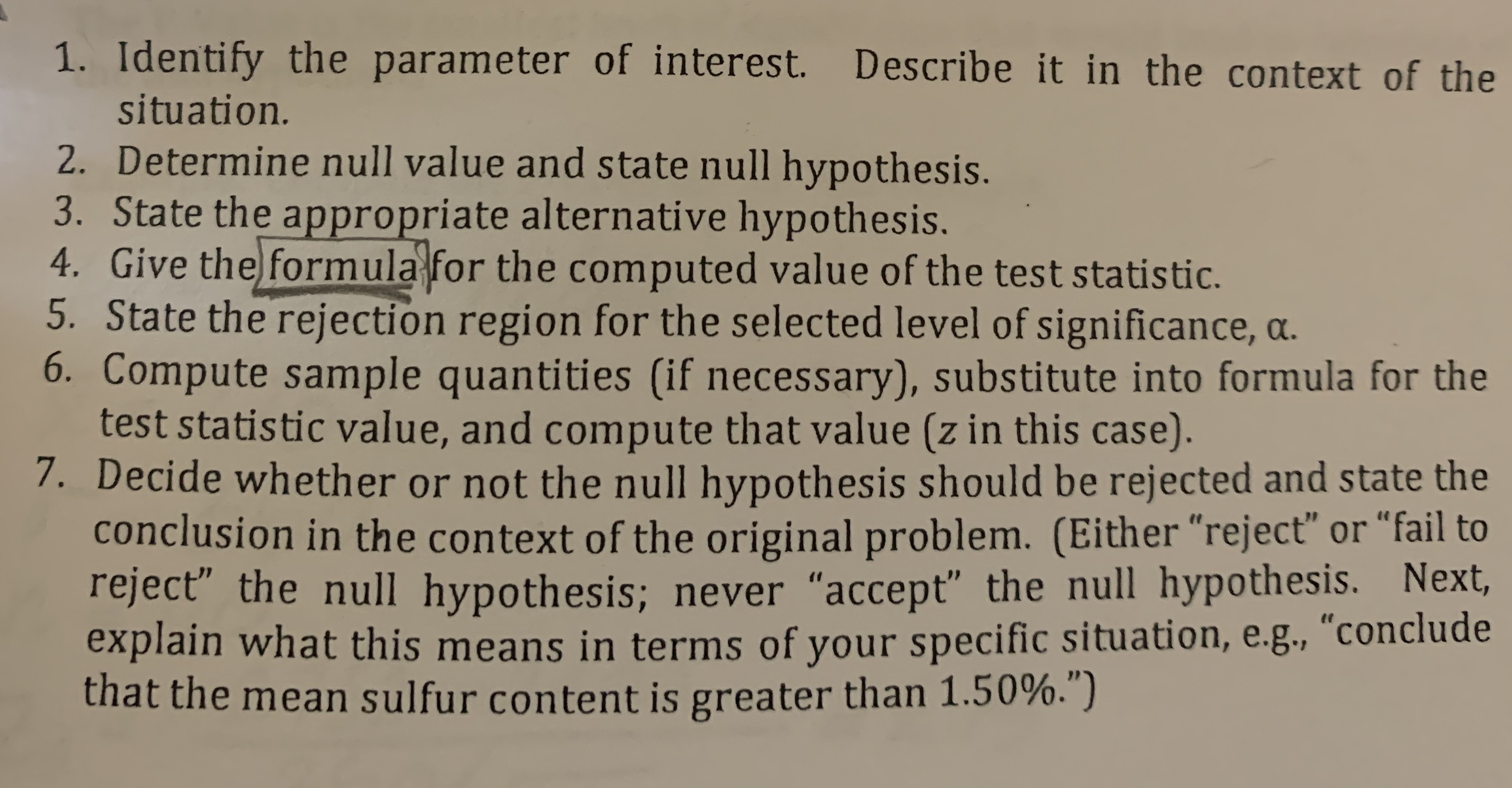# For the problems below, when asked to conduct the hypothesis test, please use the seven-stepprocess we have been using in class. Also, in step 7, it is very important to be clear in yourconclusion. There are three parts to this: evidence from your hypothesis test, the decision to rejector fail to reject the null hypothesis, and the conclusion in the context of the problem. For example,referring to the first example we did in Chapter 10, we stated the following-1.03 is not less than -1.96, nor is it greater than +1.96, so we fail to reject Ho: H- 2 0, andconclude that there is no difference in mean yield strength between the two types of roof bolts."(Here, the evidence is-1.03 is not less than -1.96, nor is it greater than +1.96The decision isWe fail to reject Ho: 41 - 42 = 0The conclusion in the context of the problem isWe conclude that there is no difference in mean yield strength between the two types ofroof bolts)7polisn operation for manufacturing interocular lenses used in the human eye following cataractsurgery. Three hundred lenses were tumble polished using the first polishing solution, and of thisnumber, 253 had no polishing-induced defects. Another 300 lenses were tumble-polished using thesecond polishing solution, and 196 lenses were satisfactory upon completion.(a) Use the seven-step hypothesis test that we have been using in class to determine if the twopolishing solutions differ. Use a= 0.01. Do not use the P-Value.(b) Conduct an appropriate confidence interval/bound to determine if the two polishing solutionsdifferTwo different types of polishing solutions are being evaluated for possible use in a tumble- 1. Identify the parameter of interest. Describe it in the context of thesituation.2. Determine null value and state null hypothesis.3. State the appropriate alternative hypothesis.4. Give the formula for the computed value of the test statistic.5. State the rejection region for the selected level of significance, a.6. Compute sample quantities (if necessary), substitute into formula for thetest statistic value, and compute that value (z in this case).7. Decide whether or not the null hypothesis should be rejected and state theconclusion in the context of the original problem. (Either "reject" or "fail toreject the null hypothesis; never "accept" the null hypothesis. Next,explain what this means in terms of your specific situation, e.g., "concludethat the mean sulfur content is greater than 1.50%.")

Question
97 views

THIS NOT GRADED ASSIGIMENT , IT IS AN OLD EXAM QUESTION.help_outlineImage TranscriptioncloseFor the problems below, when asked to conduct the hypothesis test, please use the seven-step process we have been using in class. Also, in step 7, it is very important to be clear in your conclusion. There are three parts to this: evidence from your hypothesis test, the decision to reject or fail to reject the null hypothesis, and the conclusion in the context of the problem. For example, referring to the first example we did in Chapter 10, we stated the following -1.03 is not less than -1.96, nor is it greater than +1.96, so we fail to reject Ho: H- 2 0, and conclude that there is no difference in mean yield strength between the two types of roof bolts." (Here, the evidence is -1.03 is not less than -1.96, nor is it greater than +1.96 The decision is We fail to reject Ho: 41 - 42 = 0 The conclusion in the context of the problem is We conclude that there is no difference in mean yield strength between the two types of roof bolts) 7 polisn operation for manufacturing interocular lenses used in the human eye following cataract surgery. Three hundred lenses were tumble polished using the first polishing solution, and of this number, 253 had no polishing-induced defects. Another 300 lenses were tumble-polished using the second polishing solution, and 196 lenses were satisfactory upon completion. (a) Use the seven-step hypothesis test that we have been using in class to determine if the two polishing solutions differ. Use a= 0.01. Do not use the P-Value. (b) Conduct an appropriate confidence interval/bound to determine if the two polishing solutions differ Two different types of polishing solutions are being evaluated for possible use in a tumble- fullscreenhelp_outlineImage Transcriptionclose1. Identify the parameter of interest. Describe it in the context of the situation. 2. Determine null value and state null hypothesis. 3. State the appropriate alternative hypothesis. 4. Give the formula for the computed value of the test statistic. 5. State the rejection region for the selected level of significance, a. 6. Compute sample quantities (if necessary), substitute into formula for the test statistic value, and compute that value (z in this case). 7. Decide whether or not the null hypothesis should be rejected and state the conclusion in the context of the original problem. (Either "reject" or "fail to reject the null hypothesis; never "accept" the null hypothesis. Next, explain what this means in terms of your specific situation, e.g., "conclude that the mean sulfur content is greater than 1.50%.") fullscreen
check_circle

Step 1

(a) Computation of seven-step hypothesis test:

(i) Parameter of interest:

Let p1 denote the proportion of first type of polishing solution and p2 denotes the proportion of second type of polishing solution.

(ii) Null hypothesis:

H0: p1 = p2

That is, the proportion of two polishing solutions are same.

(iii) Alternative hypothesis:

Ha: p1 ≠ p2

That is, the proportion of two polishing solutions are different.

(iv) Formula for test statistic:

The formula for the test statistic is as follows:

Step 2

(vii) Conclusion:

Here, the level of significance is 0.01.

The p-value (=0.000) is less than the significance level (=0.01).

That is, reject H0 at 1% level of...

### Want to see the full answer?

See Solution

#### Want to see this answer and more?

Solutions are written by subject experts who are available 24/7. Questions are typically answered within 1 hour.*

See Solution
*Response times may vary by subject and question.
Tagged in

### Statistics